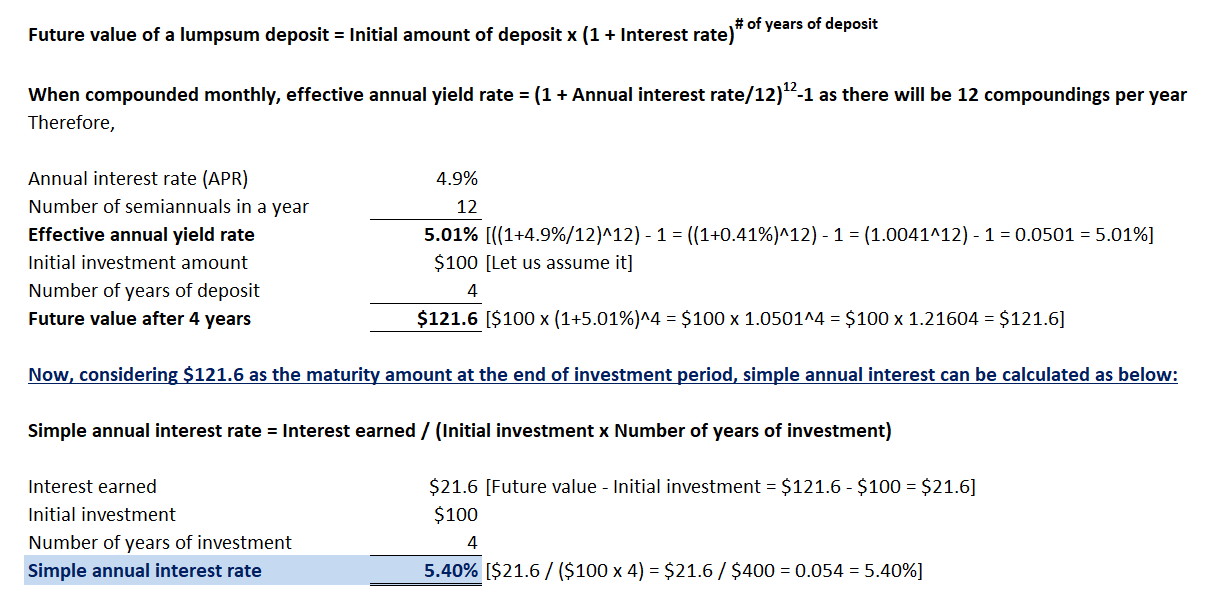In: Finance

# If money is invested for 4 years, the per annum simple interest rate equivalent to a...

If money is invested for 4 years, the per annum simple interest rate equivalent to a nominal rate of 4.9%p.a compounding monthly is (as a percentage rounded to three decimal places; don’t use % sign): Answer:

## Solutions

##### Expert Solution## Related Solutions

##### If money is invested for 10 years, the per annum simple interest rate equivalent to a...
If money is invested for 10 years, the per annum simple interest rate equivalent to a nominal rate of 6.5%p.a compounding quarterly is
##### If money is invested for 8 years at a simple interest rate of 8.6% per annum,...
If money is invested for 8 years at a simple interest rate of 8.6% per annum, the nominal interest rate per annum, compounding monthly, is (as a percentage rounded to three decimal places; no % sign):
##### An interest rate is 6.75% per annum with continuous compounding. What is the equivalent rate with...
An interest rate is 6.75% per annum with continuous compounding. What is the equivalent rate with semiannual compounding?
##### An interest rate is 7.50% per annum with annual compounding. What is the equivalent rate with...
An interest rate is 7.50% per annum with annual compounding. What is the equivalent rate with continuous compounding? (Answer is in percentage with two decimal place - example 5.35)
##### An interest rate is 9.50% per annum with continuous compounding. What is the equivalent rate with...
An interest rate is 9.50% per annum with continuous compounding. What is the equivalent rate with semiannual compounding? (Answer in percent with two decimals. Example 5.25)
##### You are investing a sum of money for 4 years. You earn a simple interest rate...
You are investing a sum of money for 4 years. You earn a simple interest rate of r = 10% for the first 2 years and j12 = 6% for the last 2 years. What is the equivalent effective annual rate of return, j, you earn over each of the 4 years? A. 7.98% B. 8.81% C. 8.07% D. 7.84%
##### Calculate the simple interest. (6 marks) Principal Rate per Annum Time Simple Interest $1356.78 8% 6... Calculate the simple interest. Principal Rate per Annum Time Simple Interest$1356.78 8% 6 years $685.43 9.25% 7 months$1595.85 7.75% 185 days Calculate the principal. Simple Interest Rate per Annum Time Principal $1275.00 8.5% 3 years$585.90 9.25% 5 months $210.75 8.75% 63 days Calculate the interest rate per annum correct to 1 decimal place. Principal Simple Interest Time Rate per Annum$10,563.77 $7394.64 7 years$6854.25 $433.53 11 months$450.00 $7.22 66 days Calculate the simple interest, then... ##### Phyllis invested$8,500, a portion earning a simple interest rate of 3 1/4% per year and...
Phyllis invested $8,500, a portion earning a simple interest rate of 3 1/4% per year and the rest earning a rate of 3% per year. After one year the total interest earned on these investments was$271.25. How much money did she invest at each rate? at 3 1/4% per year $_______ at 3% per year$________
##### A loan of RM5,000 for 20 months at 10% per annum simple interest rate was settled...
A loan of RM5,000 for 20 months at 10% per annum simple interest rate was settled by making 20 monthly payments. Find the outstanding principal just after the eighth payment by using the Rule of 78.